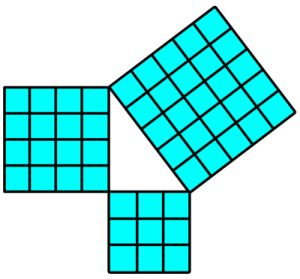# Looks like Pythagoras Theorem$\large 15x^2=7y^2+3^2$

How many integral solutions exist for the above equation?

×